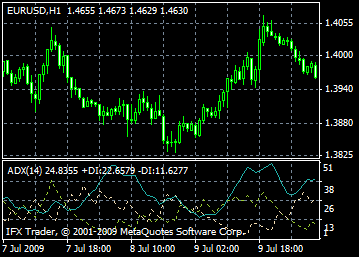# ADX indicator formula and settings

The average directional index (ADX) is a leading indicator which measures how strong the current trend is before a trade is opened and helps to determine whether the trend is going to weaken or continue. This adx indicator was developed by J. Welles Wilder and described in his book New Concepts in Technical Trading Systems.

The ADX indicator is a combination of two other indicators: 14-period positive directional indicator (+DI) and negative directional indicator (-DI). The ADX line is usually built alongside the +DI and –DI curves. The ADX consists of three lines that reflect the direction and strength of the trend:

• +DI shows upward movements
• -DI shows downward movements

If +DI is above –DI, price is moving upwards; and if –DI is above +DI, price is trending downwards.

Thus, +DI above –DI generates a buy signal; and –DI above +DI, a sell signal. If these lines cross, the trend is changing.

To reduce false signals, Wilder offered the rule of peaks, according to which one should mark the price peak hit when +DI and –DI cross. In case +DI is rising above –DI, the peak will be the price high of the day when the lines cross each other. If +DI is dropping below –DI, the peak will be an intraday low.

Later this peak is used as an entry point to the market. For example, once +D is above –DI, wait until the price breaks above the peak and only then open buy deals. If the price fails to break above the high, remain short.

The ADX indicator is the best indicator to determine the current market conditions. Use it to find out the trend strength and reap the best profits.#### Calculation